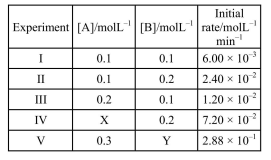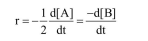# The results given in the below table were obtained during kinetic studies of the following reaction:

Question:

The results given in the below table were obtained during kinetic studies of the following reaction:

$2 \mathrm{~A}+\mathrm{B} \longrightarrow \mathrm{C}+\mathrm{D}$$X$ and $Y$ in the given table are respectively :

1. $0.3,0.4$

2. $0.4,0.3$

3. $0.4,0.4$

4. $0.3,0.3$

Correct Option: 1

Solution:

From rate law$=K[A]^{x}[B]^{y}$

$6 \times 10^{-3}=K(0.1)^{x}(0.1)^{y}$.........(1)

$2.4 \times 10^{-2}=\mathrm{K}(0.1)^{\mathrm{x}}(0.2)^{\mathrm{y}}$.........(2)

$1.2 \times 10^{-2}=\mathrm{K}(0.2)^{\mathrm{x}}(0.1)^{y}$..........(3)

$(3) \div(1) \Rightarrow x=1$

$(2) \div(3) \Rightarrow x=2$

So, other with respect to $\mathrm{A}=1$

Order with respect to $\mathrm{B}=2$

$(4) \div(3)$

$\left(\frac{\mathrm{x}}{0.2}\right) \times\left(\frac{0.2}{0.1}\right)^{2}=\frac{7.2 \times 10^{-2}}{1.2 \times 10^{-2}}$

$x=\frac{6 \times 0.2}{4}$

$\mathrm{x}=0.3 \mathrm{M}$

$(5) \div(4)$

$\left(\frac{y}{0.2}\right)^{2}=\frac{2.88 \times 10^{-1}}{7.2 \times 10^{-2}}$

$y^{2}=4 \times 0.22$

$y=0.4 \mathrm{M}$